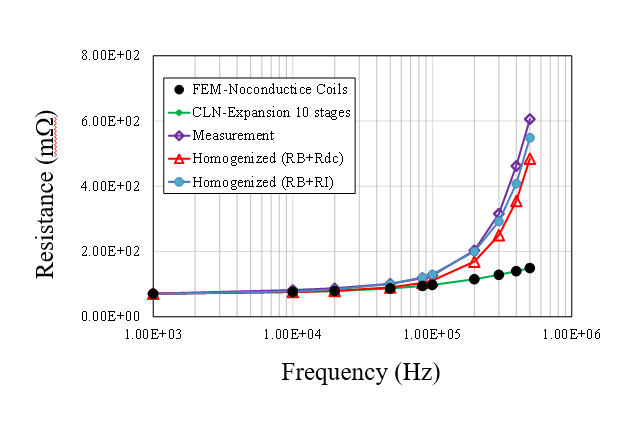# Electromagnetic field analysis software## Analysis Service by CLN Method

As a specialist in electromagnetic field analysis,
we thoroughly support your company's design issues and their verification!

### Contract analysis service utilizing SSIL's proprietary CLN method

The CLN method is an innovative method that has the following features by expressing eddy current fields as equivalent circuits (ladder circuits).

(1) The amount of calculation for eddy current analysis can be greatly reduced.
(2) The obtained equivalent circuit can be used for time-domain simulations.

In our contracted analysis service, we create and provide frequency-dependent equivalent circuits for inductors and coils using the CLN method. From the circuit simulation results using the obtained equivalent circuit, eddy currents and magnetic field distribution in transient behavior can also be obtained by post-processing the CLN method. Compared to coupled analysis with the finite element method, the speed is overwhelmingly faster.

The CLN method is very useful for linear eddy current analysis coupled with circuit simulation (induction heating, power electronics circuit analysis, etc.), Litz wire analysis, and so on.
We plan to release it as a software product in the future, but we will offer contract analysis services first, so please take advantage of it.

#### Analysis Example 1: Construction of an equivalent circuit for an inductor with frequency dependence

This is an example of creating an equivalent circuit of an inductor using the CLN method and simulating a DC-DC converter circuit using it. Since a frequency-dependent equivalent circuit can be generated, it can be applied to transient analysis. Figure 1 shows the analyzed DC-DC converter circuit. Figure 2 shows the circuit simulation results. Figure 3 shows the eddy current distribution obtained from the circuit simulation results by post-processing the CLN method.

#### Analysis Example 2: AC Loss Evaluation of Litz Wire

The following is an example of evaluating the AC resistance of a Litz wire coil for a wireless power transfer system using the coil homogenization method and the CLN method.
The evaluation are performed in the following two steps.

(1) Unit cell analysis using the CLN method
(2) Finite element AC analysis of the overall model

In the unit cell analysis, a single Litz wire is modeled and its equivalent complex permeability is obtained, taking into account its periodicity and other factors. The Biot-Savart CLN method does not require a mesh for the air region, so even complex Litz wire meshing can be easily performed using a dedicated meshing tool.
Next, the complex permeability is used to determine the AC losses of the coil using a finite element AC analysis of the overall model and the losses are converted to AC resistance.

Using this method, we evaluated the AC resistance of test coils for a wireless power transfer system.
Figure 1 shows the model of the unit cell analysis. Figure 2 shows a photograph of the Litz wire coil for testing. Figure 3 shows the overall model of the wireless power transfer system. Figure 4 compares the AC resistance obtained from the analysis of the coil with that obtained from the measurement. The calculated AC resistance of the coil agrees with the measured value within a range of about 10% up to a range of several hundred kHz.Figure 4 Frequency dependence of AC resistance of the secondary coil (comparison of analytical results and measuremenst)

Note: This analysis was conducted with support from the Acquisition, Technology & Logistics Agency's National Security Technology Research Promotion Fund